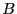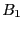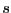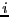Next: Hessian procedure Up: General purpose solving algorithm Previous: Single bisection mode   Contents

Implementation

The generic implementation of this solving procedure is:

int Solve_General_JH_Interval(int Dimension_Var,int Dimension_Eq,
INTEGER_VECTOR &Type_Eq,
INTERVAL_VECTOR (* TheIntervalFunction)(int,int,INTERVAL_VECTOR &),
INTERVAL_MATRIX (* Hessian)(int, int, INTERVAL_VECTOR &),
INTERVAL_VECTOR & TheDomain,
int Order,
int Iteration,
int Stop_First_Sol,
double Accuracy_Variable,
double Accuracy,
INTERVAL_MATRIX & Solution,
INTEGER_VECTOR & Is_Kanto,
int Apply_Kanto,
int (* Simp_Proc)(INTERVAL_VECTOR &),
int (* Local_Newton)(int Dimension,int Dimension_Eq,
INTERVAL_VECTOR (* TheIntervalFunction)(int,int,INTERVAL_VECTOR &),
INTERVAL_MATRIX (* Gradient)(int, int, INTERVAL_VECTOR &),
VECTOR &Input,double Accuracy,int Max_Iter, VECTOR &Residu,INTERVAL_VECTOR &In))
the arguments being:
• m: number of unknowns
• n: number of functions, see the note 2.3.4.1
• Type_Eq: type of the functions, see the note 2.3.4.2
• IntervalFunction: a function which return the interval vector evaluation of the functions, see the note 2.3.4.3. Remember that if you have equations and inequalities in the system you must first define the equations and then the inequalities.
• IntervalGradient: a function which return the interval matrix of the jacobian of the functions, see the note 2.4.2.2
• IntervalHessian: a function which return the interval matrix of the Hessian of the functions, see the note 2.5.2.1
• TheDomain: box in which we are looking for solution of the system. A copy of the search domain is available in the global variable ALIAS_Init_Domain
• Order: the type of order which is used to store the intervals created during the bisection process. This order may be either MAX_FUNCTION_ORDER or MAX_MIDDLE_FUNCTION_ORDER. See the note on the order 2.3.4.4.
• M: the maximum number of boxes which may be stored. See the note 2.5.2.2
• Stop: the possible values are 0,1,2
• 0: the algorithm will look for every solution in TheDomain
• 1: the algorithm will stop as soon as 1 solution has been found
• 2: the algorithm will stop as soon as Nb solutions have been found
• epsilon: the maximal width of the box, see the note 2.3.4.6
• epsilonf: the maximal width of the function intervals, see the note 2.3.4.6
• Solution: an interval matrix of size (Nb,m) which will contained the solution intervals. Each solution may be:
• a set of intervals with the associated flag IsKanto to 0:
• a set of intervals with the associated flag IsKanto to 1: there is an unique solution in the set and Newton method will converge toward this solution
• a set of intervals reduced to a point with the associated flag IsKanto to 0: this point is a solution which has been obtained with Krawczyk method (see 2.10). The accuracy of this solution may be improved by using the point as starting point for Krawczyk method and decreasing the accuracy epsilonf
• IsKanto: an integer vector of dimension Nb. A value of 1 for IsKanto(i) indicate that applying Newton method (see section 2.9) with as estimate the center of the solution intervals Solution(i) will converge toward the unique solution which lie within the solution intervals Solution(i)
• ApplyKanto: an integer which indicate at which level we use Kantorovitch theorem. If 1 we use Kantorovitch theorem (see section 3.1.2 and the mathematical background) to determine the solution. A consequence is that the solution interval may have a width larger than epsilon. If 0 we use Kantorovitch theorem just to separate the solutions: the solution interval will have a width epsilon. If 2 we will apply Newton method for every box which has not been eliminated during the bisection process but we will consider the result a solution only if it lie within the box. The maximal number of iteration is determined by the global variable Max_Iter_Newton_JH_Interval (by default 100). In that case we may miss solutions if they are lying inside the same box.
• Nb: the maximal number of solution which will be returned by the algorithm
• GM: an interval matrix which give a-priori information on the values of the derivatives of the function. GM(i,j) is the interval value of the derivative of function i with respect to variable j
• Simp_Proc: a user-supplied procedure that take as input the current box and proceed to some further reduction of the width of the box or even determine that there is no solution for this box, in which case it should return -1 (see note 2.3.3). Remember that you may use the 3B method to improve the efficiency of this algorithm (see section 2.3.2).
• Local_Newton: a Newton scheme that is used when Apply_Kanto is set to 2. When omitted the algorithm will use the ALIAS Newton procedure (see section 2.9).

Note that the following arguments may be omitted:

• Type_Eq: in that case all the functions will supposed to be equations.
• GM: in that case all the derivatives will supposed to be unknown
• Simp_Proc: no simplification procedure is provided by the user
• Local_Newton

The following variables play also a role in the computation:

• ALIAS_Store_Gradient: if not 0 the gradient matrix of each box will be stored together with the boxes. Must be set to 0 for large problem (default value: 1)
• ALIAS_Diam_Max_Gradient: if the maximal width of the ranges in a box is lower than this value, then the gradient will be used to perform the interval evaluation of the functions (default value: 1.e10)
• ALIAS_Diam_Max_Kraw: if the maximal width of the ranges in a box is lower than this value, then the Krawczyk operator will be used to determine if there is a unique solution in the box (default value: 1.e10)
• ALIAS_Diam_Max_Newton: if the maximal width of the ranges in a box is lower than this value, then the interval Newton method will be used either to try to reduce the width of the box or to to ensure that there is no solution of the system in the box (default value: 1.e10)
• ALIAS_Always_Use_Inflation: if ApplyKanto is set to 1 we get for each solution a boxwhich contains only one solution. If this flag is set to 1 we compute the solution using Newton and then we use an inflation procedure that try to determine a boxwhich is larger thanand contains also only one solution
• ALIAS_Eps_Inflation: the inflation algorithm will try to increase the width of the boxby at least this value
• ALIAS_Sing_Determinant: if the determinant of the jacobian matrix of the system is lower than this value, then the system is supposed to be singular
• ALIAS_Diam_Sing: for a valueof this parameter if there is singular solution in the system, then the algorithm will not look for solution of the system in a box of width 2around the singular solution (default value: 0)
• ALIAS_Use_Grad_Equation: if this integer array has a size of n the derivatives of equationwill be used to evaluate the i-th equation only if ALIAS_Use_Grad_Equation[i] is not 0
• ALIAS_No_Hessian_Evaluation: if set to 0 we will not use the Hessian to sharpen the interval evaluation of the Gradient when performing the interval evaluation of the equations. This may be useful if its known that the interval evaluation of the elements of the hessian will have always a constant sign

SubsectionsNext: Hessian procedure Up: General purpose solving algorithm Previous: Single bisection mode   Contents
Jean-Pierre Merlet 2012-12-20